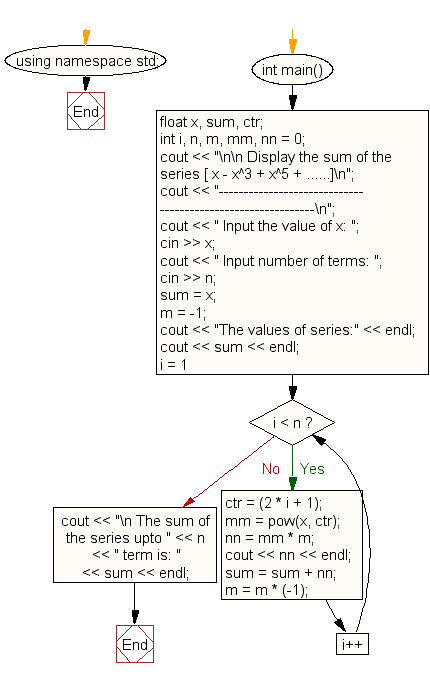﻿ C++ : Find the sum of the series [ x - x^3 + x^5 + ......]# C++ Exercises: Find the sum of the series [ x - x^3 + x^5 + ......]

## C++ Exercises: For Loop Exercise-25 with Solution

Write a program in C++ to find the sum of the series [ x - x^3 + x^5 + ......].

Sample Solution:-

C++ Code :

``````#include <iostream>
#include <math.h>
using namespace std;

int main()
{
float x, sum, ctr;
int i, n, m, mm, nn = 0;
cout << "\n\n Display the sum of the series [ x - x^3 + x^5 + ......]\n";
cout << "------------------------------------------------------------\n";
cout << " Input the value of x: ";
cin >> x;
cout << " Input number of terms: ";
cin >> n;
sum = x;
m = -1;
cout << "The values of series:" << endl;
cout << sum << endl;
for (i = 1; i < n; i++) {
ctr = (2 * i + 1);
mm = pow(x, ctr);
nn = mm * m;
cout << nn << endl;
sum = sum + nn;
m = m * (-1);
}
cout << "\n The sum of the series upto " << n << " term is: " << sum << endl;
}
``````

Sample Output:

``` Display the sum of the series [ x - x^3 + x^5 + ......]
------------------------------------------------------------
Input the value of x: 2
Input number of terms: 5
The values of series:
2
-8
32
-128
512

The sum of the series upto 5 term is: 410
```

Flowchart:C++ Code Editor:

Contribute your code and comments through Disqus.

What is the difficulty level of this exercise?

﻿